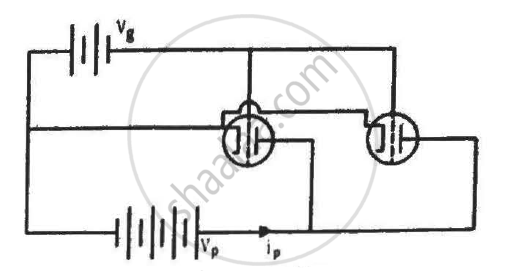Share

# The Figure Shows Two Identical Triode Tubes Connected in Parallel. the Anodes Are Connected Together and the Cathodes Are Connected Together. - Physics

ConceptTriode

#### Question

The figure shows two identical triode tubes connected in parallel. The anodes are connected together and the cathodes are connected together. Show that the equivalent plate resistance is half the individual plate resistance, the equivalent mutual conductance is double the individual mutual conductance and the equivalent amplification factor is the same as the individual amplification factor.#### Solution

From the circuit,

Cathode of both the triodes are connected to a common point and their anodes are also connected together. Thus, the 2 triodes are connected in parallel.

As plate resistance is obtained by observing the variation of plate voltage with plate current, keeping grid voltage as constant.

Hence equivalent plate resistance

$R_p = \frac{r_{P_1} \times r_{P_2}}{r_{P_1} + r_{P_2}}$

if both the triodes have equal plate resistance

$i . e . r_{P_1} = r_{P_2} = r_P$

$R_P = \frac{r_P}{2}$

To find the equivalent transconductance

We need to look at the variation of current with respect to grid voltage, keeping plate voltage as constant.

From the circuit

Now the 2 triodes are in series w.r.t grid voltage.

Hence equivalent transconductance will be equal to

$G_m = 2 g_m$

if the 2 triodes have equal trens conductance i.e $g_{m_1} = g_{m_2} = g_m$

Now the equivalent amplification factor is,

$\mu_{\text{equivalent}} = G_m \times R_P$

$\mu_{\text{equivalent}} = 2 g_m \times \frac{r_P}{2}$

$\mu_{\text{equivalent}} = \mu$

Is there an error in this question or solution?

#### APPEARS IN

Solution The Figure Shows Two Identical Triode Tubes Connected in Parallel. the Anodes Are Connected Together and the Cathodes Are Connected Together. Concept: Triode.
S